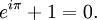## March 11, 2008

### leading up to π day...

In case you didn't know, π day is coming up. (It is 3/14... get it?) To lead into π day I thought I would throw in some random mentions of π.

The first one is from an identity called Euler's Identity. It is an equation that involves π and a few other prominent numbers. Maybe even the most prominent and important numbers. Here is that equation:Here is a clip from Wikipedia that does a pretty good explanation of this amazing equation:

Euler's identity is considered by many to be remarkable for its mathematical beauty. Three basic arithmetic operations occur exactly once each: addition, multiplication, and exponentiation. The identity also links five fundamental mathematical constants:

Furthermore, in mathematical analysis, equations are commonly written with zero on one side.

Whether you are a mathematician or not, this is amazing. the five most important numbers all in one equation with all of the basic mathematical operations... Weird.